# 具有非线性非局部边界条件的非散度型退化抛物方程的定性分析Qualitative Analysis of Nondivergent Degraded Parabolic Equations with Nonlinear Nonlocal Boundary Condition

DOI: 10.12677/PM.2019.92021, PDF, HTML, XML, 下载: 414  浏览: 595  科研立项经费支持

Abstract: In this paper, we consider the qualitative analysis of a class of degenerate parabolic equation of non-divergence type with non-linear and non-local boundary conditions. Under the condition of generalized exponential terms, the global existence and blow-up properties of solutions of the equation under various conditions are discussed by using the upper and lower solutions method.

1. 引言

$\left\{\begin{array}{l}{u}_{t}=f\left(u\right)\left(\Delta u+a{\int }_{\Omega }{u}^{\gamma }\left(x,t\right)\text{d}x\right),x\in \Omega ,t>0,\\ u\left(x,t\right)={\int }_{\Omega }g\left(x,y\right){u}^{l}\left(y,t\right)\text{d}y,x\in \partial \Omega ,t>0,\\ u\left(x,0\right)={u}_{0}\left(x\right),x\in \Omega ,\end{array}$ (1.1)

(H1) ${u}_{0}\left(x\right)\in {C}^{2+\alpha }\left(\Omega \right)\cap C\left(\stackrel{¯}{\Omega }\right),\alpha \in \left(0,1\right),{u}_{0}\left(x\right)>0,x\in \Omega$ ，且

${u}_{0}\left(x\right)={\int }_{\Omega }g\left(x,y\right){u}_{0}{}^{l}\left(y\right)\text{d}y,x\in \partial \Omega$

(H2) 当 $x\in \partial \Omega ,y\in \stackrel{¯}{\Omega }$ 时， $g\left(x,y\right)$ 是连续非负函数，且 $g\left(x,y\right)\overline{)\equiv }0$

(H3) $f\left(s\right)\in C\left(0,\infty \right)\cap {C}^{1}\left(0,\infty \right),f\left(s\right)>0,{f}^{\prime }\left(s\right)\ge 0,s\in \left(0,\infty \right)$

${u}_{t}=f\left(u\right)\left(\Delta u+a{\int }_{\Omega }u\text{d}x\right),$ (1.2)

$\phi \left(x\right)$ 是如下线性椭圆问题的唯一正解

$-\Delta \phi =1,x\in \Omega ;\phi \left(x\right)=0,x\in \partial \Omega .$ (1.3)

$\left\{\begin{array}{l}{u}_{t}=\Delta u+g\left(x,u\right),x\in \Omega ,t>0,\\ u\left(x,t\right)={\int }_{\Omega }f\left(x,y\right)u\left(y,t\right)\text{d}y,x\in \partial \Omega ,t>0,\end{array}$ (1.4)

$\left\{\begin{array}{l}{u}_{t}=f\left(u\right)\left(\Delta u+au\left({x}_{0},t\right)\right),x\in \Omega ,t>0,\\ u\left(x,t\right)={\int }_{\Omega }g\left(x,y\right)u\left(y,t\right)\text{d}y,x\in \partial \Omega ,t>0,\\ u\left(x,0\right)={u}_{0}\left(x\right),x\in \Omega ,\end{array}$ (1.5)

1) 如果 $a$ 充分小，那么问题(1.1)的解整体存在；

2) 如果 $a$ 充分大，且存在某个正数 $\delta$ 使得 ${\int }_{\delta }^{+\infty }1/\left(sf\left(s\right)\right)\text{d}s=+\infty$ ，那么问题(1.1)的解整体存在。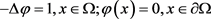(1.6)

2. 比较原理(2.1)(2.2)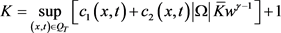，其中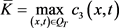。由于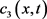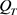内有界且连续，可知。定义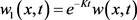，由以上变换我们易知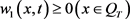。事实上，从(2.2)的第二式和第三式，利用转换式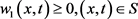。假定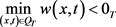，从而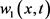的最小值在内取得。不失一般性，我们假定最小值在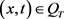处取得，从而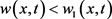对于任一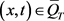带入(2.2)第一式，得到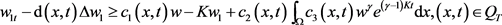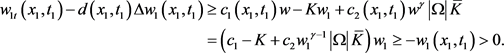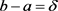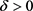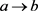，则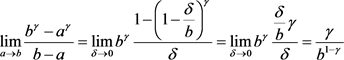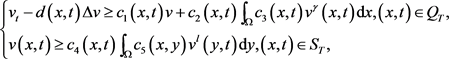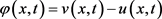，则由已知条件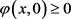，且在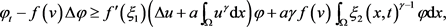3. 整体存在和有限时刻爆破

1) 设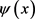是线性椭圆问题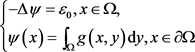(3.1)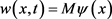， (3.2)(3.3)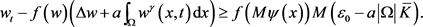(3.4)

2) 考虑常微分方程(3.5)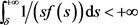，那么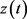整体存在且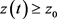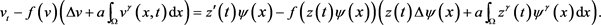(3.6. A)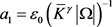，令足够大且满足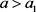，则只需取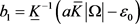(3.6. B)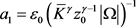，令满足，且取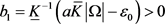，仍得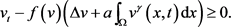(3.7)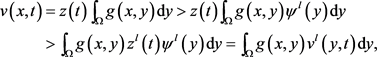(3.8)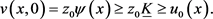(3.9)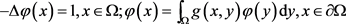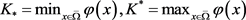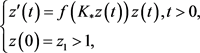(3.10)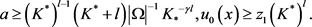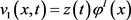(3.11)(3.12)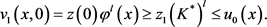(3.13)是常微分方程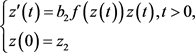(3.14)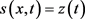，则对于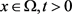，有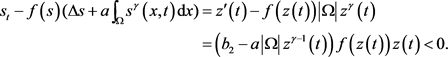(3.15)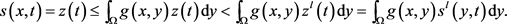(3.16)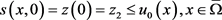. (3.17)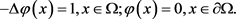是上述线性椭圆方程问题的特解。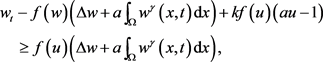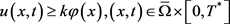。证讫。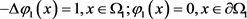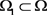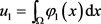，则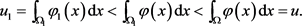(3.18)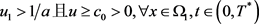.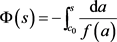,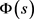严格单调递减且在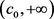上满足凸性且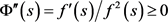。因此的反函数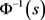存在且严格单调递减。并满足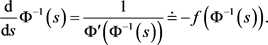(3.19)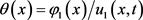。定义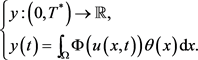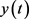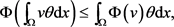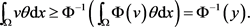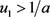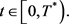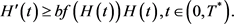(3.20)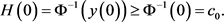(3.21)

  Deng, W., Li, Y. and Xie, C. (2003) Existence and Nonexistence of Global Solutions of Some Nonlocal Degenerate Parabolic Equa-tions. Applied Mathematics Letters, 16, 803-808. https://doi.org/10.1016/S0893-9659(03)80118-0  Friedman, A. (1986) Monotonic Decay of Solutions of Parabolic Equations with Nonlocal Boundary Conditions. Quarterly of Applied Mathematics, 44, 401-407. https://doi.org/10.1090/qam/860893  Lin, Z. and Liu, Y. (2004) Uniform Blow up Profiles for Diffusion Equations with Nonlocal Source and Nonlocal Boundary. Acta Mathematica Scientia, 24, 443-450. https://doi.org/10.1016/S0252-9602(17)30168-6  Chen, Y. (2011) Blow-Up for a localized Singular Parabolic Equation with Weighted Nonlocal Nonlinear Boundary Conditions. Journal of Mathematical Analysis & Applications, 384, 421-430. https://doi.org/10.1016/j.jmaa.2011.05.079  钟光胜. 几类非线性抛物方程(组)解的性质研究[D]: [博士学位论文]. 镇江: 江苏大学, 2016.  Deng, K. (1992) Comparison Principle for Some Nonlocal Problems. Quarterly of Applied Mathematics, 50, 517-522. https://doi.org/10.1090/qam/1178431